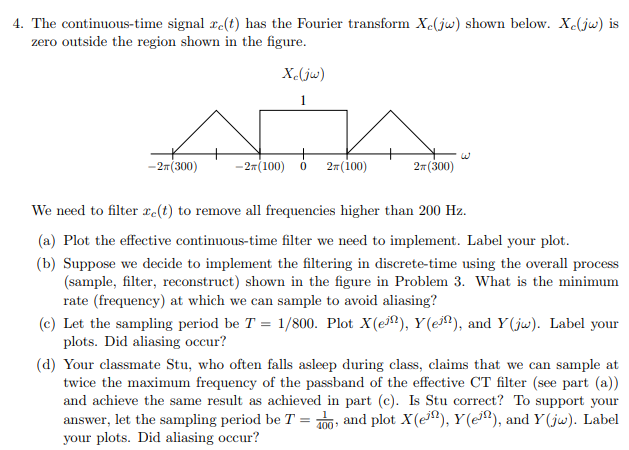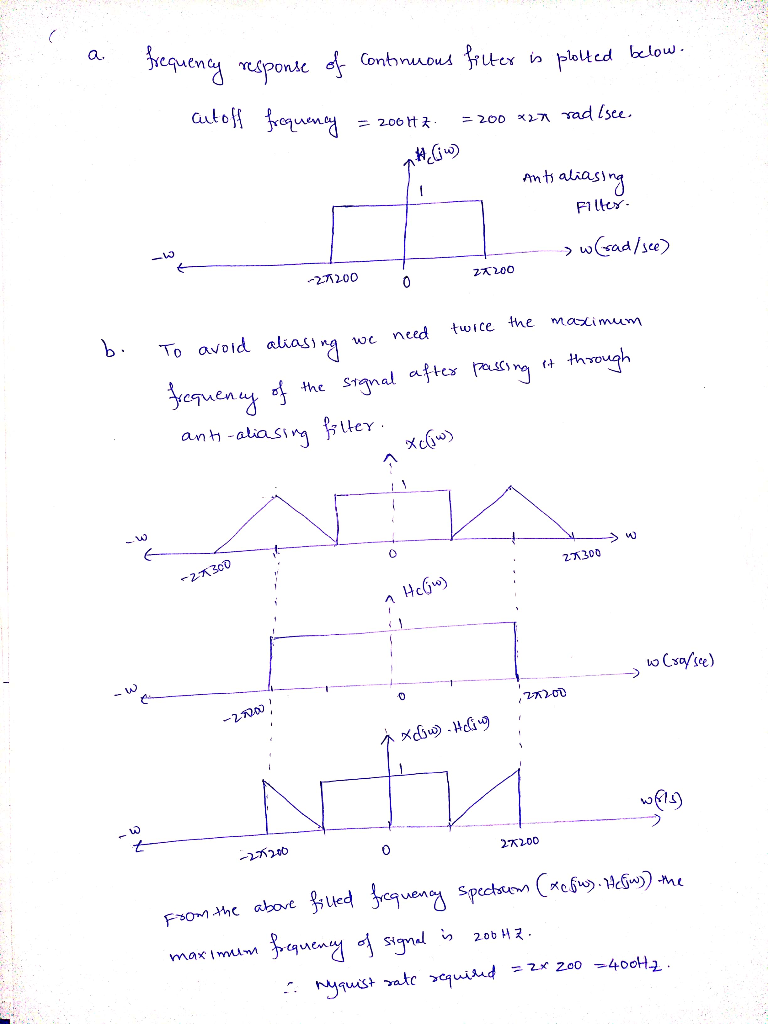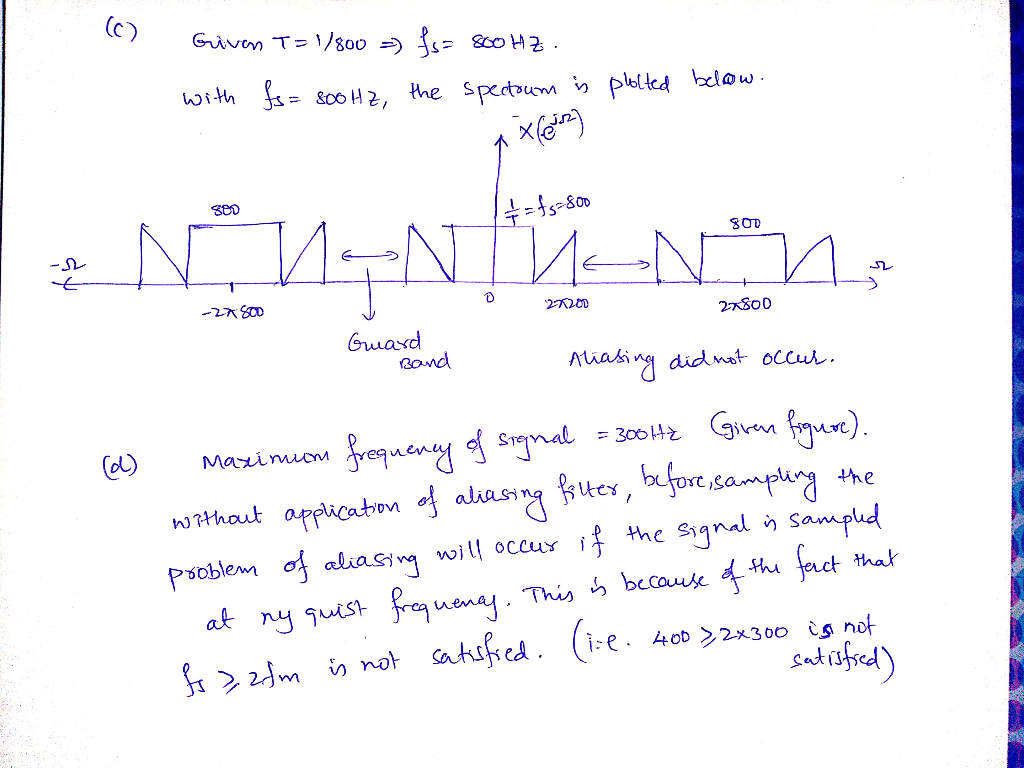Homework Help Question & Answers

# 4. The continuous-time signal e(t) has the Fourier transform X(jw) shown below. Xe(ju) is zero ou...4. The continuous-time signal e(t) has the Fourier transform X(jw) shown below. Xe(ju) is zero outside the region shown in the figure X.Gj) -2T (300) -2r(100) 0 2n(100) 2T (300) We need to filter re(t) to remove all frequencies higher than 200 Hz. (a) Plot the effective continuous-time filter we need to implement. Label your plot. b) Suppose we decide to implement the filtering in discrete-time using the overall process (sample, filter, reconstruct) shown in the figure in Problem 3. What is the minimum rate (frequency) at which we can sample to avoid aliasing? Let the sampling period be T- plots. Did aliasing occur? (c) 1/800. Plot X(eM), Y(eM), and Y(jw). Label your (d) Your classmate Stu, who often falls asleep during class, claims that we can sample at twice the maximum frequency of the passband of the effective CT filter (see part (a)) and achieve the same result as achieved in part (c). Is Stu correct? To support your answer, let the sampling period be T-100 and plot X(ejl), Y(e ), and Y(jw). Label your plots. Did aliasing occur?##### Add Answer of: 4. The continuous-time signal e(t) has the Fourier transform X(jw) shown below. Xe(ju) is zero ou...
More Homework Help Questions Additional questions in this topic.

• #### Signals and Systems. Discrete Time Fourier Transorm 2. Use the properties of the DTFT to determine the signal which has DTF1T 2. Use the properties of the DTFT to determine the signal which has...

Need Online Homework Help?

Get FREE EXPERT Answers
WITHIN MINUTES
Related Questions